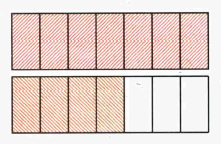Improper Fractions

Related calculators: Mixed Number to Improper Fraction Calculator , Improper Fraction to Mixed Number Calculator , Fractions Calculator

Improper Fraction is a fraction in which numerator is greater or equal than denominator. In other words improper fractions are fractions that are not proper.

Examples of improper fractions are $\frac{{5}}{{5}}$, $\frac{{8}}{{7}}$, $\frac{{125}}{{3}}$.

Fractions that are not improper are $\frac{{1}}{{5}}$, $\frac{{7}}{{19}}$, $\frac{{127}}{{128}}$.Another example of improper fraction is following.

Suppose you have two rectangles. You divide each of them into 7 parts. You paint all 7 parts of first rectangle and 4 parts of second rectangle.

In this case you paint 7+4=11 out of 7 parts: $\frac{{11}}{{7}}$.

If we paint 14 parts instead of 7 then we would obtain $\frac{{14}}{{7}}={2}$ rectangles.

Since in improper fraction numerator is greater or equal than denominator then improper fraction is greater or equal than one.